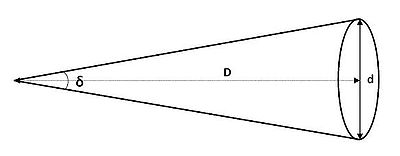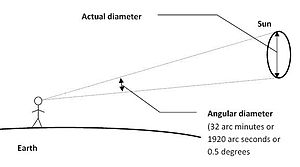Angular diameter

﻿
Angular diameter

The angular diameter or apparent size of an object as seen from a given position is the “visual diameter” of the object measured as an angle. In the vision sciences it is called the visual angle. The visual diameter is the diameter of the perspective projection of the object on a plane through its centre that is perpendicular to the viewing direction. Because of foreshortening, it may be quite different from the actual physical diameter for an object that is seen under an angle. For a disk-shaped object at a large distance, the visual and actual diameters are the same.

FormulasDiagram for the formula of the angular diameter

The angular diameter of an object can be calculated using the formula:$\delta = 2 \arctan \left( \tfrac{1}{2}\,d / D \right),$

in which δ is the angular diameter, and d and D are the visual diameter of and the distance to the object, expressed in the same units. When D is much larger than d, δ may be approximated by the formula δ = d / D, in which case the result is in radians.

For a spherical object whose actual diameter equals dact, the angular diameter can be found with the formula:$\delta = 2 \arcsin \left( \tfrac{1}{2}\,d_\mbox{act} / D \right).$

For practical use, the distinction between the visual diameter d and the actual diameter dact only makes a difference for spherical objects that are relatively close.

Use in astronomy

In astronomy the sizes of objects in the sky are often given in terms of their angular diameter as seen from Earth, rather than their actual sizes. Since these angular diameters are typically small, it is common to present them in arcseconds. An arcsecond is 1/3600th of one degree, and a radian is 180/π degrees, so one radian equals 3600.180/π arcseconds, which is about 206265 arcseconds. Therefore, the angular diameter of an object with visual diameter d at a distance D, expressed in arcseconds, is given by:

δ = 206265 d / D arcseconds.

The angular diameter of Earth's orbit around the Sun, from a distance of one parsec, is 2″ (two arcseconds).

The angular diameter of the Sun, from a distance of one light-year, is 0.03″, and that of the Earth 0.0003″. The angular diameter 0.03″ of the Sun given above is approximately the same as that of a person at a distance of the diameter of the Earth.

This table shows the angular sizes of noteworthy celestial bodies as seen from the Earth:

Celestial body Angular diameter Relative size (10 pixels per arcsecond)
Sun 31.6′ – 32.7′ 28.7 – 29.7 times the maximum value for Venus (orange bar below)
Moon 29.3′ – 34.1′ 26.6 – 31.0 times the maximum value for Venus (orange bar below)
Venus 9.565″ – 66.012″

Jupiter 29.800″ – 50.115″

Saturn 14.991″ – 20.790″

Mars 3.492″ – 25.113″

Mercury 4.535″ – 13.019″

Uranus 3.340″ – 4.084″

Neptune 2.179″ – 2.373″

Ceres 0.330″ – 0.840″

Vesta 0.20" – 0.64"

Pluto 0.063″ – 0.115″

R Doradus 0.052″ – 0.062″

Betelgeuse 0.049″ – 0.060″

Eris 0.034" – 0.089″

Alphard 0.00909″
Alpha Centauri A 0.007″
Canopus 0.006″
Sirius 0.005936″
Altair 0.003″
Deneb 0.002″
Proxima Centauri 0.001″

The table shows that the angular diameter of Sun, when seen from Earth is approximately 32 arcminutes (1920 arcseconds or 0.53 degrees), as illustrated above.Angular diameter : the angle subtended by an object

This meaning the angular diameter of the Sun is about 250,000 that of Sirius (it has twice the diameter and the distance is 500,000 times as much; the Sun is 10,000,000,000 times as bright, corresponding to an angular diameter ratio of 100,000, so Sirius is roughly 6 times as bright per unit solid angle).

The angular diameter of the Sun is also about 250,000 that of Alpha Centauri A (it has the same diameter and the distance is 250,000 times as much; the Sun is 40,000,000,000 times as bright, corresponding to an angular diameter ratio of 200,000, so Alpha Centauri A is a little brighter per unit solid angle).

The angular diameter of the Sun is about the same as that of the Moon (the diameter is 400 times as large and the distance also; the Sun is 200,000 to 500,000 times as bright as the full Moon (figures vary), corresponding to an angular diameter ratio of 450 to 700, so a celestial body with a diameter of 2.5 to 4″ and the same brightness per unit solid angle would have the same brightness as the full Moon).

Even though Pluto is physically larger than Ceres, when viewed from Earth, e.g. through the Hubble Space Telescope, Ceres has a much larger apparent size.

While angular sizes measured in degrees are useful for larger patches of sky (in the constellation of Orion, for example, the three stars of the belt cover about 3 degrees of angular size), we need much finer units when talking about the angular size of galaxies, nebulae or other objects of the night sky.

Degrees, therefore, are subdivided as follows:

• 360 degrees (°) in a full circle
• 60 arc-minutes (′) in one degree
• 60 arc-seconds (″) in one arc-minute

To put this in perspective, the full moon viewed from earth is about ½ degree, or 30 arc minutes (or 1800 arc-seconds). The moon's motion across the sky can be measured in angular size: approximately 15 degrees every hour, or 15 arc-seconds per second. A one-mile-long line painted on the face of the moon would appear to us to be about one arc-second in length.

In astronomy, it is typically difficult to directly measure the distance to an object. But the object may have a known physical size (perhaps it is similar to a closer object with known distance) and a measurable angular diameter. In that case, the angular diameter formula can be inverted to yield the Angular diameter distance to distant objects as$d \equiv 2 D \tan \left ( \frac{\delta}{2} \right)$.

In non-Euclidean space, such as our expanding universe, the angular diameter distance is only one of several definitions of distance, so that there can be different "distances" to the same object. See Distance measures (cosmology).

Non-circular objects

Many deep sky objects such as galaxies and nebulas are non-circular and are thus typically given two measures of diameter: Major Diameter and Minor Diameter. For example, the Small Magellanic Cloud has a visual apparent diameter of 5° 20′ × 3° 5′.

Defect of illumination

Defect of illumination is the maximum angular width of the unilluminated part of a celestial body seen by a given observer. For example, if an object is 40 seconds of arc across and is 75 percent illuminated, the defect of illumination is 10 seconds of arc.

Wikimedia Foundation. 2010.

Look at other dictionaries:

• angular diameter — kampinis skersmuo statusas T sritis fizika atitikmenys: angl. angular diameter; apparent diameter vok. scheinbare Durchmesser, m; Winkeldurchmesser, m rus. видимый диаметр, m; угловой диаметр, m pranc. diamètre angulaire, m; diamètre apparent, m …   Fizikos terminų žodynas

• angular diameter — Astron. the angle that the apparent diameter of a celestial object subtends at the eye of the observer. [1870 75] * * * …   Universalium

• angular diameter — /ˌæŋgjələ daɪˈæmətə/ (say .anggyuhluh duy amuhtuh) noun the angle which the apparent diameter of a celestial body subtends at the observer s eye …   Australian English dictionary

• angular diameter — Astron. the angle that the apparent diameter of a celestial object subtends at the eye of the observer. [1870 75] …   Useful english dictionary

• Angular diameter distance — The angular diameter distance is a distance measure used in astronomy. The angular diameter distance to an object is defined in terms of the object s actual size, x, and heta the angular size of the object as viewed from earth. d A= frac{x}{… …   Wikipedia

• Angular resolution — describes the resolving power of any image forming device such as an optical or radio telescope, a microscope, a camera, or an eye. Definition of terms Resolving power is the ability of the components of an imaging device to measure the angular… …   Wikipedia

• Angular size redshift relation — The angular size redshift relation describes the relation between the angular size observed on the sky of an object of given physical size, and the objects redshift from Earth (which is related to its distance, d , from Earth). In a Euclidean… …   Wikipedia

• Diameter — For the notion of diameter in graph theory, see Distance (graph theory). For the computer network protocol, see Diameter (protocol). Diameter In geometry, a diameter of a circle is any straight line segment that passes through the center of the… …   Wikipedia

• angular aperture — n the angle subtended at the principal focus of an optical system (as a microscope) by the diameter of its entrance for light …   Medical dictionary

• Angular aperture — The angular aperture of a lens is the apparent angle of the lens aperture as seen from the focal point::a = 2 arctan left( frac {D/2} {f} ight)where :f is the focal length:D is the diameter of the aperture. Relation to numerical aperture In a… …   Wikipedia